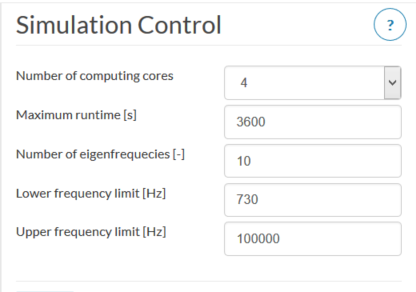# Eigenmode in Harmonic Simulation

I did the RepRap simulation and started a second harmonic simulation in which I tried to simulate the Eigenmode after the first 10 modes. In the setup the lowes frequency was the 10th mode from the first simulation and I searched for 10 modes. I got only one mode at the the 10th mode. I figured out I have to increase the numbers of modes I am searching for.
OK. If I didn’t have done a simulation from 0Hz and know how many modes exist between 0Hz and the frequency I am interested in how should I know how many modes I have to search for? I recommend that the numbers of modes should be for the frequency band I am run the simulation.

Hello @sburger, thanks for the feedback!

@afischer, maybe you can take a further look into it.

Hey @sburger,

I am not sure if I understand this correctly, so I try to wrap it up again. You are running a harmonic analysis where you try to figure out the harmonic response for a frequency range that contains 10 eigenfrequencies starting fromt the 10th eigenfrequency/eigenmode right?

I guess you are referring to your setup “Harmonische Anregung 3” with a frequency range of 50 Hz starting at 690 Hz with a frequency step of 10 Hz. As I said I am not sure if I got you correctly so I am not sure if I answer now to the right thing. The frequency stepping only defines the resolution of the defined frequency range. Typically you get high peaks at the eigenfrequencies (magnitude depending on the harmonic load case), so if your frequency stepping is pretty big it is most likely to not show those peaks correctly as the frequency steps would need to hit coincidentally the eigenfrequency. It does not mean that it searches for 10 eigenfrequencies. That is why the frequency analysis prior to the harmonic analysis makes so much sense as you can then define the frequency range and resolution. Otherwise it is not possible to know that. For an industrial use case the frequency range that you are interested in will be probably known e.g. the frequency range an engine passes through during a start-up process where you want to make sure that the bearing does not fail/break because of eigenfrequency modes are induced.

Hope that helps!

Best Alex

Sorry, I didn’t describe it right.

I was talking about the Eigenmode in Frequency analysis.
Let say I have a resonance around 3.3kHz. At the moment I have to set up the analysis from 0Hz up to 3.5kHz with, I don’t know, 1000 modes. If I am lucky the number of modes will be sufficient. If I set up the analysis from 3kHz to 3.5kHz with 50 modes it will be without a result because in this case there are 731 modes before the mode around 3.3kHz.

I recommend that the numbers of modes in the frequncy analysis should be for the frequency band I am run the simulation.

Hey @sburger,

I do not understand why a simulation where you calculate the 50 lowest modes between 3kHz and 3.5kHz should be without a result?

Let’s take the 3D-printer project as an example. If you calculate for example the first 10 eigenfrequencies/eigenmodes it will look like this (copied from your project):

You can see that there are the 7th and 8th right below 700 Hz and 2 modes above 700 Hz. If you are interested in frequencies above 600 Hz, you can for example run the same analysis changing the interval of interest to 600 up to some very high number if you definitely want to get 20 results. Then the solver calculates the 20 lowest eigenfrequencies that are above the limit of 600 Hz. If there are more than 20 in the interval, the rest will be disregarded. See below:

(the 4 first eigenfrequencies are matching to the last result + the 16 next frequencies).

If you would define a range which contains less than the specified max number (20 in this case) of frequencies, the result will contain only those eigenfrequencies.

(only two frequencies between 600 and 700Hz)

Hope it is clearer now!

Best Alex

1 Like

This wasn’t happen with my simulation. As I wrote I had the interval from 730Hz up to 10000Hz and it should analyse 10 modes. I got only one mode as a result. I changed the numbers of modes up to 20 and got the additional modes.

To be sure I will do the simulation again. Maybe I did change two settings.

Hi @sburger,

Could your frequency step be too course to capture these modes?

Just a thought.
Christopher

Ok. It doen.
Settings:Simulation result

ONly one modeChange settings numbers of modes = 20

Tata I got my modesAt the frequency analysis is no setting for the freqency steps. This is in the harmonic analysis.

Hi @afischer
As you can see on my replay above it is as I have described.

Hey @sburger,

this is strange, I can reproduce it as well. I did not test with 10 before. We are looking into that. Thanks for pointing it out and sorry for the confusion, as 20 worked fine I thought it was a misunderstanding. But there is definitely an issue.

Best Alex